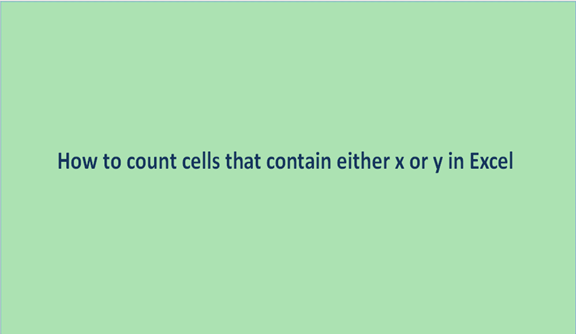## How to count cells that contain either x or y in Excel

The act of tallying a certain quantity to obtain or to reveal the number of items or several objects in a set is what we mostly refer to as counting. Counting is general and done in almost all aspects of life or our daily routines.

Counting the cells that contain either x or y is just doing the count of cells with values not necessarily x or y. The values represented in x or y can be either name of places or names of objects. When we create data in an excel sheet, we may have columns that have data that relate to one another, for example, city- Lagos or names of fruits.

These types of cells with such values are the ones we need to count and establish the value of the count in numbers. In most cases, we count in numbers that are to mean we use numbers to identify the number of items or the number of objects. Counting is done everywhere even when using excel sheets. In excel sheets we do the count of the number of rows, columns, cells, items in the rows, and columns and even the number of empty cells in an excel sheet is also counted.

The following are some of the steps to follow when you need to count the cells that contain either x or y with their examples in picture form.

Step 1

On an empty excel sheet enter the data values in cells as follows below.Step 2

Having the data set created in the above step 1, now we apply formulas to help us get the count of values of either x and y.

In this case, the values of either x or y will be juice and pie respectively. To start, we look at the function COUNTIF. This function will help us in the count of the number of cells with values juice and pie.

We incorporate the function into the function as shown. We will use the formula, =COUNTIF (A2:A7,"*juice*") + COUNTIF (A2:A7,"*pie*"). Write the formula on the formula bar and click on the enter button.